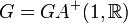# Rationally powered not implies nilpotent

This article gives the statement and possibly, proof, of a non-implication relation between two group properties. That is, it states that every group satisfying the first group property (i.e., rationally powered group) need not satisfy the second group property (i.e., nilpotent group)
View a complete list of group property non-implications | View a complete list of group property implications
Get more facts about rationally powered group|Get more facts about nilpotent group

## Statement

It is possible to construct a rationally powered group (i.e., a group that is uniquely$p$-divisible for all primes$p$) that is not a nilpotent group.

## Proof

Further information: GAPlus(1,R)

The easiest example is the following group$G = GA^+(1,\R)$:$G$ is the group of all (affine) linear maps from$\R$ to$\R$ with positive leading coefficient, under composition. Explicitly:$G = \{ x \mapsto ax + b \mid a,b \in \R, a > 0 \}$

Then, we have that:

•$G$ is rationally powered: See GAPlus(1,R) is rationally powered
•$G$ is not nilpotent: In fact, GAPlus(1,R) is centerless